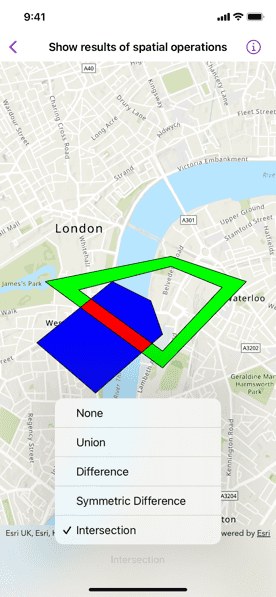# Show result of spatial operations

Find the union, intersection, or difference of two geometries.## Use case

The different spatial operations (union, difference, symmetric difference, and intersection) can be used for a variety of spatial analyses. For example, government authorities may use the intersect operation to determine whether a proposed road cuts through a restricted piece of land such as a nature reserve or a private property.

When these operations are chained together, they become even more powerful. An analysis of food deserts within an urban area might begin by union-ing service areas of grocery stores, farmers markets, and food co-ops. Taking the difference between this single geometry of all services areas and that of a polygon delineating a neighborhood would reveal the areas within that neighborhood where access to healthy, whole foods may not exist.

## How to use the sample

The sample provides an option to select a spatial operation. When an operation is selected, the resulting geometry is shown in red.

## How it works

1. Create a `GraphicsOverlay` object.
2. Create `Graphic` instances for the overlapping polygons and result.
3. Add the overlapping polygons and result graphics to the graphics overlay.
4. Perform spatial relationships between the polygons by using the appropriate operation:
• `static GeometryEngine.union(_:_:)` - This method returns the two geometries united together as one geometry.
• `static GeometryEngine.difference(_:_:)` - This method returns the difference between two geometries.
• `static GeometryEngine.symmetricDifference(_:_:)` - This method returns any part of two geometries that do not intersect.
• `static GeometryEngine.intersection(_:_:)` - This method returns the intersection of two geometries.
5. Use the geometry that is returned from the method call to update the geometry of the results graphic.

## Relevant API

• Geometry
• GeometryEngine
• Graphic
• GraphicsOverlay
• static GeometryEngine.difference(::)
• static GeometryEngine.intersection(::)
• static GeometryEngine.symmetricDifference(::)
• static GeometryEngine.union(::)

## Tags

analysis, combine, difference, geometry, intersection, merge, polygon, union

## Sample Code

ShowResultOfSpatialOperationsView.swift
Use dark colors for code blocksCopy

``````// Copyright 2022 Esri
//
// you may not use this file except in compliance with the License.
// You may obtain a copy of the License at
//
//
// Unless required by applicable law or agreed to in writing, software
// WITHOUT WARRANTIES OR CONDITIONS OF ANY KIND, either express or implied.
// See the License for the specific language governing permissions and

import SwiftUI
import ArcGIS

struct ShowResultOfSpatialOperationsView: View {
/// The current spatial operation performed.
@State private var spatialOperation = SpatialOperation.none

/// A map with a topographic basemap style and initial viewpoint.
@StateObject private var map = makeMap()

/// A graphics overlay for the map view.
@StateObject private var graphicsOverlay = makeGraphicsOverlay()

/// A graphic representing the result of the spatial operation.
private var resultGraphic: Graphic {
return graphicsOverlay.graphics.last!
}

/// An enum of spatial operations.
private enum SpatialOperation: CaseIterable {
case intersection, symmetricDifference, difference, union, none

/// A human-readable label for each spatial operation.
var label: String {
switch self {
case .none: return "None"
case .union: return "Union"
case .difference: return "Difference"
case .symmetricDifference: return "Symmetric Difference"
case .intersection: return "Intersection"
}
}
}

/// Creates a map.
private static func makeMap() -> Map {
let map = Map(basemapStyle: .arcGISTopographic)
map.initialViewpoint = Viewpoint(
center: Point(x: -13453, y: 6710127, spatialReference: .webMercator),
scale: 30_000
)
return map
}

/// Creates the graphics overlay.
private static func makeGraphicsOverlay() -> GraphicsOverlay {
// Creates the graphics for the two polygons and result.
let polygonOneGraphic = Graphic(
geometry: .polygon1,
symbol: SimpleFillSymbol(color: .blue, outline: .simple)
)

let polygonTwoGraphic = Graphic(
geometry: .polygon2,
symbol: SimpleFillSymbol(color: .green, outline: .simple)
)

let resultGraphic = Graphic(
symbol: SimpleFillSymbol(color: .red, outline: .simple)
)

// Adds the graphics to the overlay.
return GraphicsOverlay(graphics: [polygonOneGraphic, polygonTwoGraphic, resultGraphic])
}

/// Updates the result graphic based on the spatial operation.
private func performOperation() {
let resultGeometry: Geometry?
// Updates the geometry based on the selected spatial operation.
switch spatialOperation {
case .none:
resultGeometry = nil
case .union:
resultGeometry = GeometryEngine.union(.polygon1, .polygon2)
case .difference:
resultGeometry = GeometryEngine.difference(.polygon1, .polygon2)
case .symmetricDifference:
resultGeometry = GeometryEngine.symmetricDifference(.polygon1, .polygon2)
case .intersection:
resultGeometry = GeometryEngine.intersection(.polygon1, .polygon2)
}
// Updates the result graphic geometry.
resultGraphic.geometry = resultGeometry
}

var body: some View {
MapView(map: map, graphicsOverlays: [graphicsOverlay])
.onChange(of: spatialOperation) { _ in
performOperation()
}
.toolbar {
ToolbarItem(placement: .bottomBar) {
Picker("Spatial Operation", selection: \$spatialOperation) {
ForEach(SpatialOperation.allCases, id: \.self) { operation in
Text(operation.label)
}
}
}
}
}
}

private extension LineSymbol {
/// A solid, thin, black line.
static var simple: LineSymbol {
SimpleLineSymbol(style: .solid, color: .black, width: 1)
}
}

private extension Geometry {
/// The geometry for polygon one.
static var polygon1: Geometry {
Polygon(
points: [
Point(x: -13960, y: 6709400),
Point(x: -14660, y: 6710000),
Point(x: -13760, y: 6710730),
Point(x: -13300, y: 6710500),
Point(x: -13160, y: 6710100)
],
spatialReference: .webMercator
)
}

/// The geometry for polygon two.
static var polygon2: Geometry {
Polygon(
parts: [
// The outer ring.
MutablePart(
points: [
Point(x: -13060, y: 6711030),
Point(x: -12160, y: 6710730),
Point(x: -13160, y: 6709700),
Point(x: -14560, y: 6710730),
Point(x: -13060, y: 6711030)
],
spatialReference: .webMercator
),
// The inner ring.
MutablePart(
points: [
Point(x: -13060, y: 6710910),
Point(x: -14160, y: 6710630),
Point(x: -13160, y: 6709900),
Point(x: -12450, y: 6710660),
Point(x: -13060, y: 6710910)
],
spatialReference: .webMercator
)
]
)
}
}``````